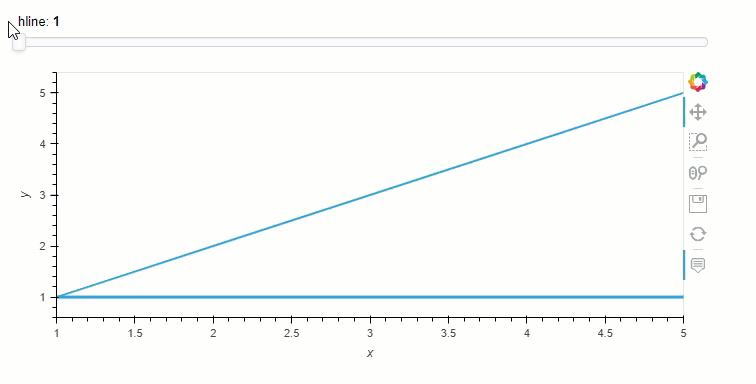# How do I link the position of a HLine to a widget?

I have a `panel.widgets.DiscreteSlider` object, and would like the vertical position of a `holoviews.HLine` to depend on the value of the widget (to be overlayed on a 2D plot for the purposes of indicating the position of a 1D slice, both generated by the `hvplot.interactive` API). Just passing the widget as the `y` parameter of the `HLine` raises a `ValueError`, and passing the widget’s value gives a static `HLine` that doesn’t respond to changes.

This feels like something utterly trivial, but I can’t seem to figure it out from the docs.

1 Like

One way to do this would be with Panel and `pn.bind`/ `pn.depends`.``````import pandas as pd

import hvplot.pandas
import holoviews as hv

value = pd.DataFrame({"x": [1, 2, 3, 4, 5], "y": [1,2,3,4,5]})

plot = value.hvplot(x="x", y="y")

def hline(value):
return hv.HLine(value)

import panel as pn
pn.extension()

slider = pn.widgets.IntSlider(value=1, start=1, end=5, name="hline")
hline = pn.bind(hline, slider)

pn.Column(slider, plot*hv.DynamicMap(hline)).servable()
``````

Thanks Marc, your expertise is always appreciated. Realising that I had to explicitly wrap it in a DynamicMap directed me to the streams API and a way to get around using `pn.bind` too.

Unfortunately, this doesn’t actually work for my use case because if you replace `plot = value.hvplot(x="x", y="y")` with `plot = value.interactive.hvplot(x="x", y="y")`, it raises:

``````Exception: Nesting a DynamicMap inside a DynamicMap is not supported. Ensure that the DynamicMap callback returns an Element or (Nd)Overlay. If you have applied an operation ensure it is not dynamic by setting dynamic=False.
``````

But this is a whole separate issue, which I don’t have the bandwith to start wrapping my head around. I think I’ll just forgo having a slice indicator for now.

1 Like